# Finding Missing Numbers in a Number Sequence

Finding Missing Numbers in a Number Sequence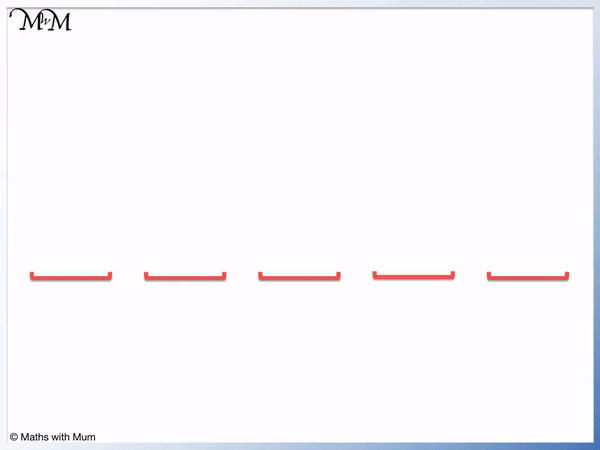• A number sequence is a list of numbers that follow a pattern.
• An example of a number sequence is 5, 9, 13, 17.
• To find the difference between each number, subtract one number from the number that comes after it.
• 9 – 5 = 4 and so, the common difference is 4.
• Each number in a sequence is called a term.
• To find the next term in the sequence, add 4 to 17 to make 21.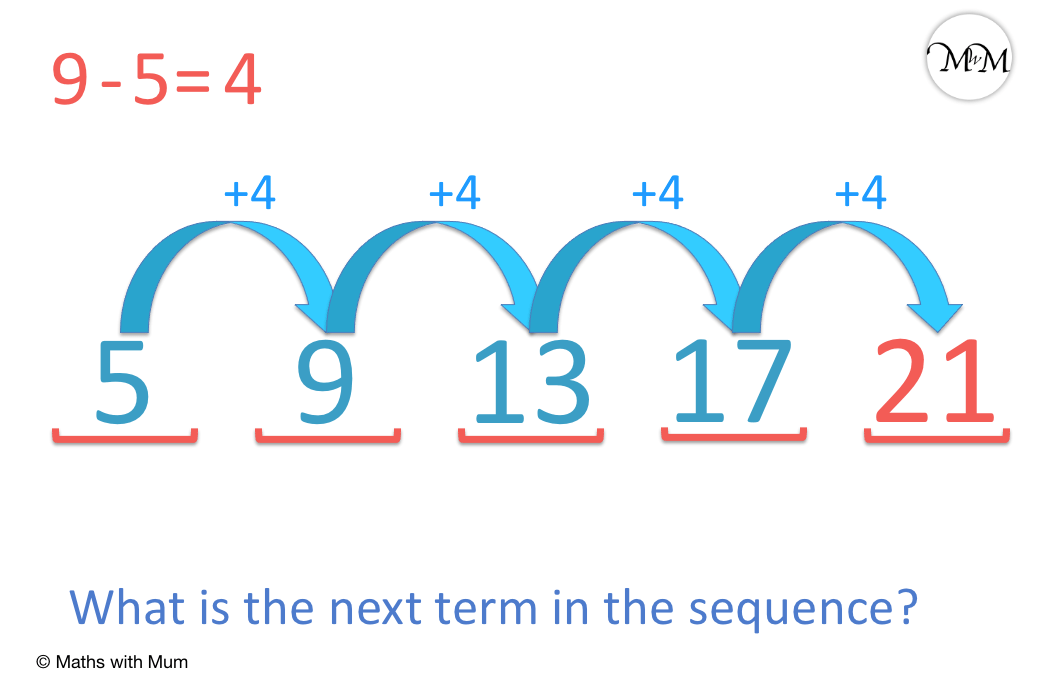A sequence can be formed by adding the same number each time.

Subtract one number from the number that comes after it to find this common difference.• To find the difference between each number, we subtract one term from the term that is immediately after it.
• 32 – 22 = 10 and so, this sequence is going up by 10 each time.
• We can add 10 to 32 to find the missing number of 42.
• Alternatively, we can subtract 10 from 52 to find 42.Supporting Lessons

#### How to Find the Common Difference in a Number Sequence# Number Sequences

## What is a Number Sequence?

A number sequence is a pattern of numbers formed using a rule. For example, 1, 4, 7, 10 is a number sequence that is formed by starting at 1 and adding 3 each time. We can add 3 more to find the next number in the sequence, which is 13.

Each number in a sequence is called a term.

A number sequence is made up of different terms. The example below shows a sequence made up of 5 terms.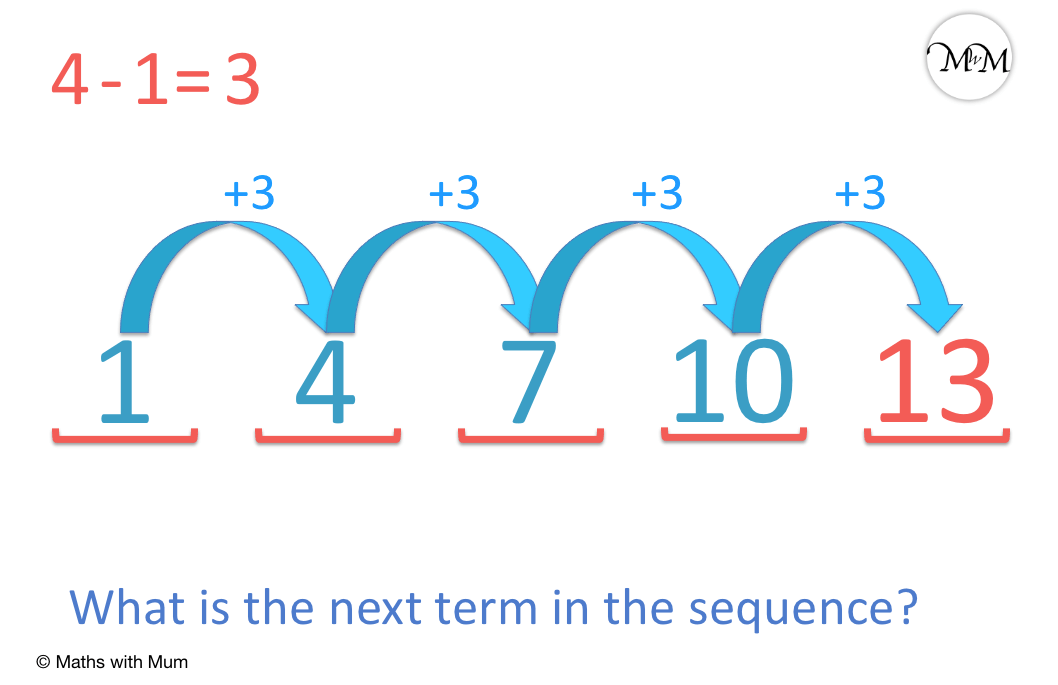An arithmetic sequence is a number pattern made by adding or subtracting the same amount to get from one number to the next. For example, in the sequence of 1, 4, 7, 10, 13, we add 3 to get from one number to the next.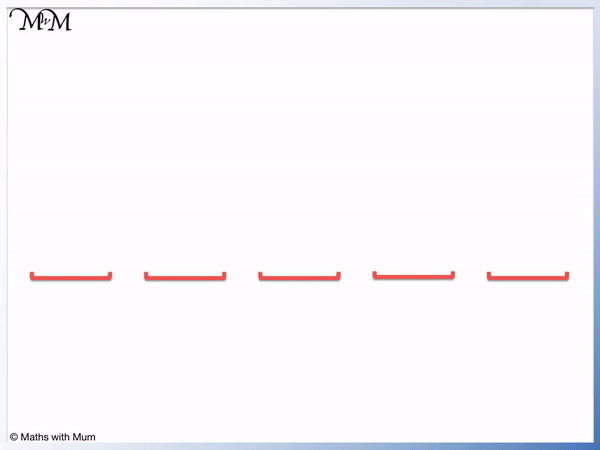To write a number sequence, write each term separated by a commma.

Some examples of basic number sequences are the times tables. The sequence formed by the 2 times table begins 2, 4, 6, 8, 10.The two times table goes up in twos each time. We can say that we add 2 to get from one term to the next.## How to Find the Next Number in a Sequence

To find the next number in a sequence, first find the common difference by subtracting one term from the term that comes immediately after it. Add this common difference on to the last term in the sequence to find the next number in the sequence.

For example, in the sequence, 3, 5, 7, 9, the difference from one term to the next is 2. To find the next number in the sequence, add 2 to 9 to make 11.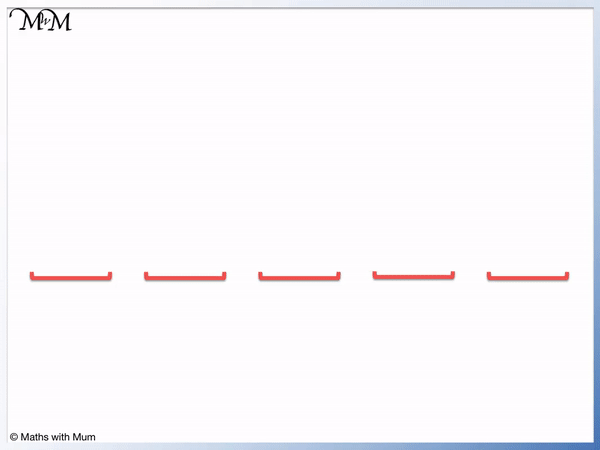We can find the common difference by subtracting any two numbers that are next to each other. 5 – 3 = 2 and 9 – 7 = 2. The common difference is 2.

We add 2 to get from each number to the next.Here is an example of finding the next number in a sequence.The first step is to find the common difference.

We subtract one term from the term that comes after it.

9 – 5 = 4 and so, the common difference is 4.We add 4 on to the final number in the sequence to find the next term.

17 + 4 = 21 and so, the missing term in this sequence is 21.

We can continue to add 4 on to continue the sequence. We would get the following sequence: 5, 9, 13, 17, 21, 25, 29, 33, 37, 41, 45, 59, 63 and so on.

## How to Find a Missing Term in an Arithmetic Sequence

To find a missing term in an arithmetic sequence, first identify the common difference by subtracting any term from the term that comes immediately after it. Count up or down by this amount from term to term until you find the missing value needed.

An arithmetic sequence is simply a list of numbers that are formed by adding on the same amount each time to get from one number to the next.

Here is the sequence 3, _, 7, 9, 11. The second term is missing.To find the common difference, we subtract any term from the term that comes after it.

11 – 9 = 2 and 9 – 7 = 2. We can see that the common difference is 2.We can add 2 to 3 to find the missing term of 5.

Alternatively, we can subtract 2 from 7 to find the missing term of 5.

Here is an example of finding 2 missing terms in a sequence.

We have the sequence _, 10, _, 24, 31.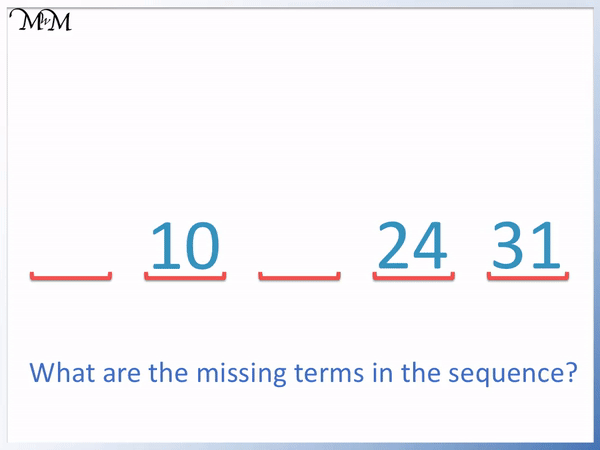We first need to find the common difference between two terms. We need to look at two consecutive terms, which are two numbers in the sequence that are next to each other.31 – 24 = 7 and so, the common difference of this sequence is 7.

We can count down in sevens to find the missing terms.

24 – 7 = 17 and 10 – 7 = 3. 17 and 3 are the missing terms in the sequence.

## How to Find the Common Difference of a Sequence

The common difference is simply the difference between each term in a sequence. To find the common difference, subtract one term from the term that comes after it. If there are no two consecutive terms, then find the difference between any two terms and divide this by the number of jumps between these terms.

For example, here is the sequence 10, _, _, _, 50.The difference between 50 and 10 is 40. We divide this difference by the number of jumps between the two terms.There are 4 jumps between the terms of 10 and 50. Therefore we divide the difference of 40 by 4 to share it evenly over these 4 jumps.

40 ÷ 4 = 10 and so the common difference is 10.

We can add on in tens to find the missing terms.

The completed sequence is 10, 20, 30, 40, 50.

Here is another example of working out the common difference in an arithmetic sequence.

We have 2, _, 10, _, _.

The two known terms are 10 and 2.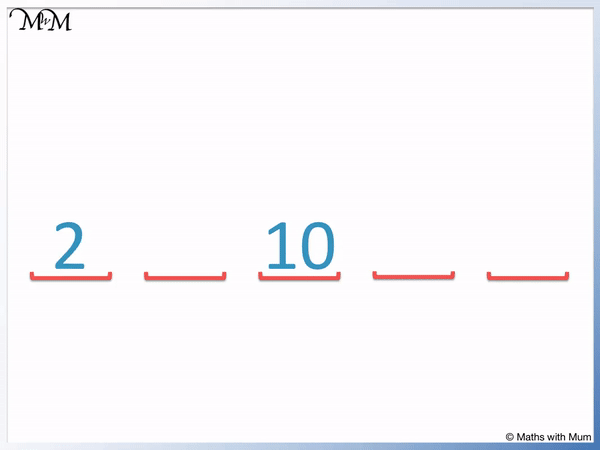10 – 2 = 8 and so, the difference between the terms is 8. However this difference needs to be shared over two jumps.

8 ÷ 2 = 4 and so, each jump is worth an addition of 4.

We add on in fours to get from one term to the next.The completed sequence is 2, 6, 10, 14, 18.

Here is another example of finding the missing terms in a sequence when we only have 2 known terms.

We have _, 7, _, _, 28.

The difference between 28 and 7 is 21.This difference of 21 is shared equally over 3 jumps.

21 ÷ 3 = 7. The common difference is 7.

We can add on in sevens to find the missing terms.The sequence is 0, 7, 14, 21, 28.

## How to Find the nth Term of a Sequence

To find the nth term of a sequence, follow these steps:

1. Find the difference from one term to the next in the sequence.
2. Multiply this difference by ‘n’ and write this down.
3. Decide what number must be added to or subtracted from the difference found in step 1 in order to make the first term of the sequence.
4. Add this number to the end of the expression written in step 2.

The nth term is a formula that is used to create a sequence. Simply substitute values in place of n to find the relevent term. To find the first term, substitute n = 1. To find the second term, substitute n = 2.

Here is the 2n sequence.The 2n sequence begins with 2, 4, 6, 8 and so on.

The 2n sequence is the two times table. The difference between each number is 2.

Here is an example of finding the nth term of the sequence 2n + 3.

The sequence is 5, 7, 9, 11.

The first step is to find the common difference between each term. This term is going up in twos.

The second step is to write this difference multiplied by n. We have 2n.The third step is to decide what number should be added to this difference to make the first term.

The difference is 2 and the first term is 5. We need to add 3 to 2 to make 5.

The final step is to write this on the end of the expression. 2n becomes 2n + 3.

We can see that the 2n + 3 sequence goes up in twos like the 2n sequence, however all numbers are 3 larger.

Here is another example of finding the nth term of a sequence.

We have 3, 8 13, 18.

The difference between each term is 5. So we start with the 5n sequence.

We need to subtract 2 from 5 to make the first term of 3 and so the sequence is 5n – 2.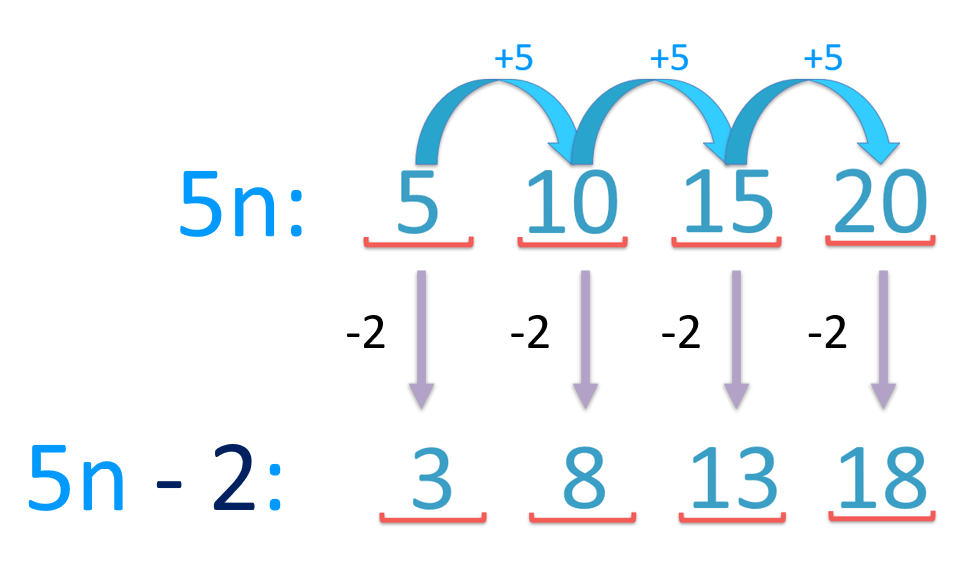The 5n sequence is the 5 times table and so, it goes up in fives each time.

The 5n – 2 sequence also goes up in fives each time but the numbers are all 2 smaller than the 5 times table.Now try our lesson on Writing and Forming Algebraic Expressions where we introduce algebraic expressions.error: Content is protected !!